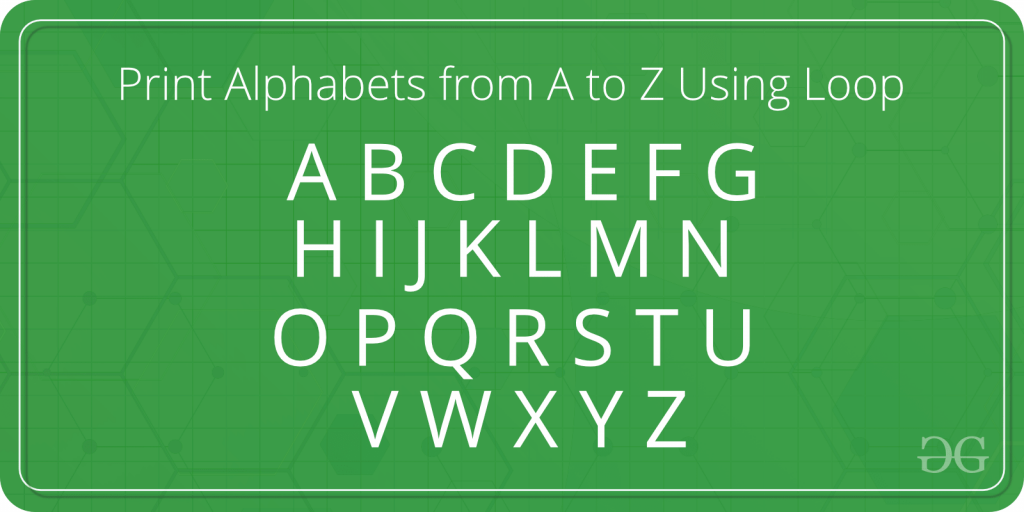# Program to Print Alphabets from A to Z Using Loop

• Difficulty Level : Basic
• Last Updated : 16 Jun, 2021The task is to print the alphabets from A to Z using a loop.

Hey! Looking for some great resources suitable for young ones? You've come to the right place. Check out our self-paced courses designed for students of grades I-XII

Start with topics like Python, HTML, ML, and learn to make some games and apps all with the help of our expertly designed content! So students worry no more, because GeeksforGeeks School is now here!

In the below program, for loop is used to print the alphabets from A to Z. A loop variable is taken to do this of type ‘char’. The loop variable ‘i’ is initialized with the first alphabet ‘A’ and incremented by 1 on every iteration. In the loop, this character ‘i’ is printed as the alphabet.

Program:

## C++14

 `// C++ program to find the print``// Alphabets from A to Z``#include ``using` `namespace` `std;` `int` `main()``{``    ``// Declare the variables``    ``char` `i;` `    ``// Display the alphabets``    ``cout << ``"The Alphabets from A to Z are: \n"``;` `    ``// Traverse each character``    ``// with the help of for loop``    ``for` `(i = ``'A'``; i <= ``'Z'``; i++)``    ``{` `        ``// Print the alphabet``        ``cout << i <<``" "``;``    ``}` `    ``return` `0;``}` `// This code is contributed by``// Shubhamsingh10`

## C

 `// C program to find the print``// Alphabets from A to Z` `#include ` `int` `main()``{``    ``// Declare the variables``    ``char` `i;` `    ``// Display the alphabets``    ``printf``(``"The Alphabets from A to Z are: \n"``);` `    ``// Traverse each character``    ``// with the help of for loop``    ``for` `(i = ``'A'``; i <= ``'Z'``; i++) {` `        ``// Print the alphabet``        ``printf``(``"%c "``, i);``    ``}` `    ``return` `0;``}`

## Java

 `// Java program to find the print``// Alphabets from A to Z``class` `GFG``{` `    ``public` `static` `void` `main(String[] args)``    ``{``        ``// Declare the variables``        ``char` `i;` `        ``// Display the alphabets``        ``System.out.printf(``"The Alphabets from A to Z are: \n"``);` `        ``// Traverse each character``        ``// with the help of for loop``        ``for` `(i = ``'A'``; i <= ``'Z'``; i++)``        ``{` `            ``// Print the alphabet``            ``System.out.printf(``"%c "``, i);``        ``}` `    ``}``}` `/* This code contributed by PrinciRaj1992 */`

## Python3

 `# Python3 program to find the print``# Alphabets from A to Z` `if` `__name__ ``=``=` `'__main__'``:``    ` `    ``# Declare the variables``    ``i ``=` `chr``;` `    ``# Display the alphabets``    ``print``(``"The Alphabets from A to Z are: "``);` `    ``# Traverse each character``    ``# with the help of for loop``    ``for` `i ``in` `range``(``ord``(``'A'``), ``ord``(``'Z'``) ``+` `1``):` `        ``# Print the alphabet``        ``print``(``chr``(i), end``=``" "``);``        ` `# This code is contributed by Rajput-Ji`

## C#

 `// C# program to find the print``// Alphabets from A to Z``using` `System;` `class` `GFG``{` `    ``public` `static` `void` `Main(String[] args)``    ``{``        ``// Declare the variables``        ``char` `i;` `        ``// Display the alphabets``        ``Console.Write(``"The Alphabets from A to Z are: \n"``);` `        ``// Traverse each character``        ``// with the help of for loop``        ``for` `(i = ``'A'``; i <= ``'Z'``; i++)``        ``{` `            ``// Print the alphabet``            ``Console.Write(``"{0} "``, i);``        ``}` `    ``}``}` `// This code is contributed by Rajput-Ji`

## Javascript

 ``

Output:

```The Alphabets from A to Z are:
A B C D E F G H I J K L M N O P Q R S T U V W X Y Z ```

My Personal Notes arrow_drop_up